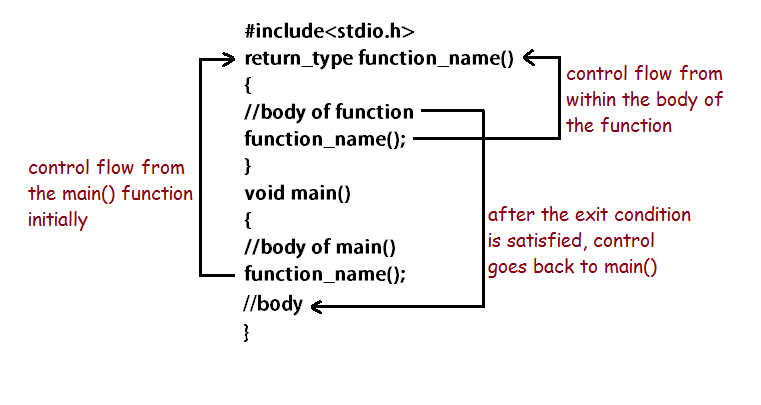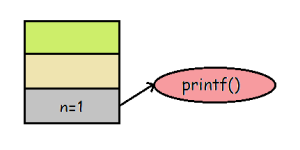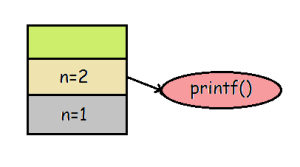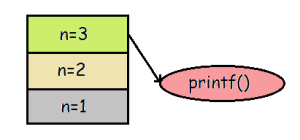# Recursive Functions with Examples in C Language

Recursion is a process of calling a function within the same function again and again till the condition is satisfied. We have already seen how functions can be declared, defined and called. Recursive functions are declared and defined in the same manner. But they are called within its own body except for the first call which is obviously made by an external method.

This way of calling the methods/functions allows a function to be executed repeatedly without the use of loops. As for stopping the repeat process, a condition can be specified by the programmer. Without an exit condition, the function would get executed repeatedly in the same manner as an infinite loop. Recursive functions need to be called by any other function first in order to start the execution process. After the exit condition is satisfied the control flows out of the function and back to the calling function. Here is how the control flows in a recursive program:### How Stack is used in Recursion?

Stack is a data structure used to implement recursion. Stack is implemented where the storage and sequence of execution of recursive program comes. Let us understand it better with an example:

```#include <stdio.h>

void recursion(int n) {
if (n <= 3) { // exit condition for recursive function
printf("%d\n", n);
recursion(++n); // calling itself again
} else {
return;
}
}

int main() {
int x = 1;
recursion(x);
return 0;
}

```

In this program, we see that the value passed to the function is 1. So in the beginning, the value of n is 1. So for the first execution, the value of n is stored at the base of the stack as we see in the figure below. Then it prints the value of n.Once the compiler encounters the call inside of the function, the function starts executing again with the updated value n=2. It gets stored as shown in the figure below:The value of n is then printed and the control flows to the calling of the function again with n=3.And the process happens again for n=4. But if the condition becomes false and it returns the control to the main() function.

This is used so as to maintain the data of every call. For example, when the call for n=3 will finish, it will return to its calling function which is actually the function itself but with another instance of it running. So when it returns back to function execution with n=2, data of n=3 execution is pop from the stack and now the data for n=2 is at the top which is loaded into the memory for the further execution of the method.

### Factorial Program Using Recursion in C Language

Here’s a simple program which prints the factorial of n numbers starting from 1 using a recursive function:

```#include <stdio.h>

int factorial(int x) // function definition
{
if (x == 1 || x == 0) { // condition on which the exit of the recursion
// depends
return 1;
} else {
return (x * factorial(x - 1)); // function being called within its own body
}
}

int main() {
int n, i, f = 1;

printf("Enter the range: \n");
scanf("%d", &n);

for (i = 1; i <= n; i++) {
f = factorial(i); // function call at the initial stages
printf("The factorial of %d is: %d \n", i, f);
}

return 0;
}

```
```Output:-
Enter the range:
5
The factorial of 1 is: 1
The factorial of 2 is: 2
The factorial of 3 is: 6
The factorial of 4 is: 24
The factorial of 5 is: 120
```

An investment in knowledge always pays the best interest. Hope you like the tutorial. Do come back for more because learning paves way for a better understanding.

Do not forget to share and Subscribe.

Happy coding!! 🙂

### Recommended -

Subscribe
Notify of